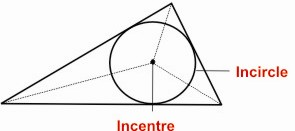HOME MATH DICTIONARY DOWNLOAD FEEDBACK DISCLAIMER
 Question: What is an Incentre and Incircle ? Answer: The three lines which bisect the angles of a triangle all meet at a point inside the triangle. This point is the same distance from each side of the triangle and so a circle can be drawn with its center at this point touching the sides of the triangle. This point is called the incentre and circle is called the incircle.Example of an Incentre and Incircle: